Want to share your content on R-bloggers? click here if you have a blog, or here if you don't.

A natural technique to select variables in the context of generalized linear models is to use a stepŵise procedure. It is natural, but contreversial, as discussed by Frank Harrell  in a great post, clearly worth reading. Frank mentioned about 10 points against a stepwise procedure.

• It yields R-squared values that are badly biased to be high.
• The F and chi-squared tests quoted next to each variable on the printout do not have the claimed distribution.
• The method yields confidence intervals for effects and predicted values that are falsely narrow (see Altman and Andersen (1989)).
• It yields p-values that do not have the proper meaning, and the proper correction for them is a difficult problem.
• It gives biased regression coefficients that need shrinkage (the coefficients for remaining variables are too large (see Tibshirani (1996)).
• It has severe problems in the presence of collinearity.
• It is based on methods (e.g., F tests for nested models) that were intended to be used to test prespecified hypotheses.
• Increasing the sample size does not help very much (see Derksen and Keselman (1992)).
• It allows us to not think about the problem.
• It uses a lot of paper.

In order to illustrate that issue of variable selection, consider a dataset I’ve been using many times on the blog,

```MYOCARDE=read.table(
"http://freakonometrics.free.fr/saporta.csv",

where we have observations from people entering E.R., because of a (potential) infarctus, and we want to understand who did survive, and to build a predictive model.

What if we use a forward stepwise logistic regression here? I want to use a forward construction since it usually yields to models with less explanatory variables. We can use Akaike Information Criterion

```> reg0=glm(PRONO~1,data=MYOCARDE,family=binomial)
> reg1=glm(PRONO~.,data=MYOCARDE,family=binomial)
> step(reg0,scope=formula(reg1),
+ direction="forward",k=2)                  # AIC
Start:  AIC=98.03
PRONO ~ 1

Df Deviance    AIC
+ REPUL  1   46.884 50.884
+ INSYS  1   51.865 55.865
+ INCAR  1   53.313 57.313
+ PRDIA  1   78.503 82.503
+ PAPUL  1   82.862 86.862
+ PVENT  1   87.093 91.093
<none>       96.033 98.033
+ FRCAR  1   94.861 98.861

Step:  AIC=50.88
PRONO ~ REPUL

Df Deviance    AIC
+ INCAR  1   44.530 50.530
+ PVENT  1   44.703 50.703
+ INSYS  1   44.857 50.857
<none>       46.884 50.884
+ PAPUL  1   45.274 51.274
+ PRDIA  1   46.322 52.322
+ FRCAR  1   46.540 52.540

Step:  AIC=50.53
PRONO ~ REPUL + INCAR

Df Deviance    AIC
<none>       44.530 50.530
+ PVENT  1   43.134 51.134
+ PRDIA  1   43.772 51.772
+ INSYS  1   44.305 52.305
+ PAPUL  1   44.341 52.341
+ FRCAR  1   44.521 52.521

Call:  glm(formula = PRONO ~ REPUL + INCAR, family = binomial, data = MYOCARDE)

Coefficients:
(Intercept)        REPUL
1.633668    -0.003564
INCAR
1.618479

Degrees of Freedom: 70 Total (i.e. Null);  68 Residual
Null Deviance:	    96.03
Residual Deviance: 44.53 	AIC: 50.53```
```> step(reg0,scope=formula(reg1),
+ direction="forward",k=log(n))           # BIC
Start:  AIC=98.11
PRONO ~ 1

Df Deviance    AIC
+ REPUL  1   46.884 51.043
+ INSYS  1   51.865 56.024
+ INCAR  1   53.313 57.472
+ PRDIA  1   78.503 82.662
+ PAPUL  1   82.862 87.021
+ PVENT  1   87.093 91.252
<none>       96.033 98.113
+ FRCAR  1   94.861 99.020

Step:  AIC=51.04
PRONO ~ REPUL

Df Deviance    AIC
+ INCAR  1   44.530 50.768
+ PVENT  1   44.703 50.942
<none>       46.884 51.043
+ INSYS  1   44.857 51.095
+ PAPUL  1   45.274 51.512
+ PRDIA  1   46.322 52.561
+ FRCAR  1   46.540 52.778

Step:  AIC=50.77
PRONO ~ REPUL + INCAR

Df Deviance    AIC
<none>       44.530 50.768
+ PVENT  1   43.134 51.452
+ PRDIA  1   43.772 52.089
+ INSYS  1   44.305 52.623
+ PAPUL  1   44.341 52.659
+ FRCAR  1   44.521 52.838

Call:  glm(formula = PRONO ~ REPUL + INCAR, family = binomial, data = MYOCARDE)

Coefficients:
(Intercept)        REPUL
1.633668    -0.003564
INCAR
1.618479

Degrees of Freedom: 70 Total (i.e. Null);  68 Residual
Null Deviance:	    96.03
Residual Deviance: 44.53 	AIC: 50.53```

With those two approaches, we have the same story: the most important variable (or say with the highest predictive value) is REPUL. And we can improve the model by adding INCAR. And that’s it. We can get a good model with those two covariates.

Now, what about using cross-validation here? We should keep in ming that AIC is asymptotically equivalent to One-Leave-Out Cross Validation (see Stone (1977)),  while BIC is equivalent to -fold Cross Validation (see Shao (1997)), where

• Using Leave-One-Out Cross Validation

In order to select the first variable, consider 7 logistic regression, each on a single different variable. Each time, we estimate the model on  observations and get a prediction on the remaining one,

on. Set . The function to get those values is

```> name_var=names(MYOCARDE)
> pred_i=function(i,k){
+ fml = paste(name_var,"~",name_var[k],sep="")
+ reg=glm(fml,data=MYOCARDE[-i,],family=binomial)
+ predict(reg,newdata=MYOCARDE[i,],
+ type="response")
+ }```

then for each variable , we get the ROC curve using and,

```> library(AUC)
> ROC=function(k){
+  Y=MYOCARDE[,8]=="Survival"
+  S=Vectorize(function(i) pred_i(i,k))
+ (1:length(Y))
+  R=roc(S,as.factor(Y))
+  return(list(roc=cbind(R\$fpr,R\$tpr),
+              auc=AUC::auc(R)))
+ }```

Here, for each variable, we compute the area under the curve (AUC criterion)

```> AUC=rep(NA,7)
> for(k in 1:7){
+   AUC[k]=ROC(k)\$auc
+   cat("Variable ",k,"(",name_var[k],") :",
+   AUC[k],"n") }
Variable  1 ( FRCAR ) : 0.4934319
Variable  2 ( INCAR ) : 0.8965517
Variable  3 ( INSYS ) : 0.909688
Variable  4 ( PRDIA ) : 0.7487685
Variable  5 ( PAPUL ) : 0.7134647
Variable  6 ( PVENT ) : 0.6584565
Variable  7 ( REPUL ) : 0.9154351```

But we can also visualize those curves,

```> plot(0:1,0:1,col="white",xlab="",ylab="")
> for(k in 1:7)
+ lines(ROC(k)\$roc,type="s",col=CL[k])
> legend(.8,.45,name_var,col=CL,lty=1,cex=.8)```(there is no PRONO here, there is a typo  in the Legend)

where here colors were obtained using

```> library(RColorBrewer)
> CL=brewer.pal(8, "Set1")[-7]```

Here ROC curves were obtained using a Leave-one-Out strategy. And the best variable (if we should keep one, and one only) is

```> k0=which.max(AUC)
> name_var[k0]
 "REPUL"```

Now, consider a stepwise procedure: we keep that ‘best’ variable in our model, and we try to add another one.

```> pred_i=function(i,k){
+ vk=c(k0,k)
+ fml = paste(name_var,"~",paste(name_var[vk],
+ collapse="+"),sep="")
+ reg=glm(fml,data=MYOCARDE[-i,],family=binomial)
+ predict(reg,newdata=MYOCARDE[i,],
+ type="response")
+ }
> library(AUC)
> ROC=function(k){
+ Y=MYOCARDE[,8]=="Survival"
+ S=Vectorize(function(i) pred_i(i,k))
+ (1:length(Y))
+ R=roc(S,as.factor(Y))
+ return(list(roc=cbind(R\$fpr,R\$tpr),
+ auc=AUC::auc(R)))
+ }
> plot(0:1,0:1,col="white",xlab="",ylab="")
> for(k in (1:7)[-k0]) lines(ROC(k)\$roc,type="s",col=CL[k])
> segments(0,0,1,1,lty=2,col="grey")
> legend(.8,.45,
+   name_var[-k0],
+   col=CL[-k0],lty=1,cex=.8)```We were already quite good. And we might expect to find another variable that will increase the predictive power of our classifier.

```> AUC=rep(NA,7)
> for(k in (1:7)[-k0]){
+  AUC[k]=ROC(k)\$auc
+  cat("Variable ",k,"(",name_var[k],") :",
+  AUC[k],"n")
+ }
Variable  1 ( FRCAR ) : 0.9064039
Variable  2 ( INCAR ) : 0.9195402
Variable  3 ( INSYS ) : 0.9187192
Variable  4 ( PRDIA ) : 0.9137931
Variable  5 ( PAPUL ) : 0.9187192
Variable  6 ( PVENT ) : 0.9137931```

And, of course, we can move foreward, add another variable, etc,

```> k0=c(k0,which.max(AUC))

> pred_i=function(i,k){
+   vk=c(k0,k)
+   fml = paste(name_var,"~",paste(
+ name_var[vk],collapse="+"),sep="")
+ reg=glm(fml,data=MYOCARDE[-i,],family=binomial)
+ predict(reg,newdata=MYOCARDE[i,],
+ type="response")
+ }
> library(AUC)
> ROC=function(k){
+  Y=MYOCARDE[,8]=="Survival"
+  S=Vectorize(function(i) pred_i(i,k))
+  (1:length(Y))
+  R=roc(S,as.factor(Y))
+  return(list(roc=cbind(R\$fpr,R\$tpr),
+  auc=AUC::auc(R)))
+ }
>
> plot(0:1,0:1,col="white",xlab="",ylab="")
> for(k in (1:7)[-k0]) lines(ROC(k)\$roc,type="s",col=CL[k])
> segments(0,0,1,1,lty=2,col="grey")
> legend(.8,.45,name_var[-k0],
+ col=CL[-k0],lty=1,cex=.8)```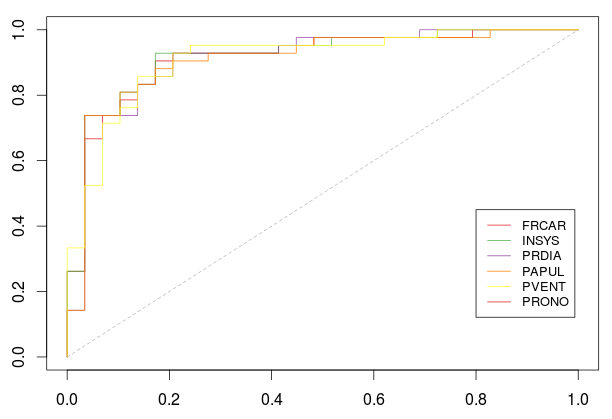But here, the gain is rather small (if any)

```> AUC=rep(NA,7)
> for(k in (1:7)[-k0]){
+ AUC[k]=ROC(k)\$auc
+ cat("Variable ",k,"(",name_var[k],") :",
+ AUC[k],"n")
+ }
Variable  1 ( FRCAR ) : 0.9121511
Variable  3 ( INSYS ) : 0.9170772
Variable  4 ( PRDIA ) : 0.910509
Variable  5 ( PAPUL ) : 0.907225
Variable  6 ( PVENT ) : 0.909688```

With that stepwise algorithm, the best strategy is to keep, first, REPUL, and then to add INCAR. Which is consistent with the stepwise procedure using Akaike Information Criterion.

An alternative could be to select the best pair among all possible pairs. It will be time consuming, but it can be used to avoid the stepwise drawback.

```> pred_i=function(i,k){
+ fml = paste(name_var,"~",paste(name_var[
+ as.integer(k)],collapse="+"),sep="")
+ reg=glm(fml,data=MYOCARDE[-i,],family=binomial)
+ predict(reg,newdata=MYOCARDE[i,],
+ type="response")
+ }
> library(AUC)
> ROC=function(k){
+   Y=MYOCARDE[,8]=="Survival"
+   L=list()
+   n=length(Y)
+   nk=trunc(n/trunc(n/10))
+   for(i in 1:(nk-1)) L[[i]]=((i-1)*
+     trunc(n/10)+1:(n/10))
+   L[[nk]]=((nk-1)*trunc(n/10)+1):n
+   S=unlist(Vectorize(function(i)
+     pred_i(L[[i]],k))(1:nk))
+   R=roc(S,as.factor(Y))
+   return(AUC::auc(R))
+ }

> v=data.frame(k1=rep(1:7,each=7),k2=rep(1:7,7))
> v=v[v\$k1<v\$k2,]
> v\$auc=NA
> for(i in 1:nrow(v)) v\$auc[i]=ROC(v[i,1:2])
> v
k1 k2       auc
2   1  2 0.9047619
3   1  3 0.9047619
4   1  4 0.6990969
5   1  5 0.6395731
6   1  6 0.6334154
7   1  7 0.8817734
10  2  3 0.9072250
11  2  4 0.9088670
12  2  5 0.8940887
13  2  6 0.8801314
14  2  7 0.8899836
18  3  4 0.8916256
19  3  5 0.8817734
20  3  6 0.9014778
21  3  7 0.8768473
26  4  5 0.6925287
27  4  6 0.7138752
28  4  7 0.8825944
34  5  6 0.6912972
35  5  7 0.8834154
42  6  7 0.8834154```

Here the best pair is

```> v[which.max(v\$auc),]
k1 k2      auc
11  2  4 0.908867
> name_var[as.integer(v[which.max(v\$auc),1:2])]
 "INCAR" "PRDIA"```

which is different, compared with the one we got above. What is odd here is that we get a smaller AUC than the ones we got at step 2 in the stepwise procedure.

Nevertheless, even with a few observations (our dataset is rather small here), it is time consuming to look at all ROC curves, for all pairs. An alternative might be to use Fold Cross Validation.

• Using -Fold Cross Validation

Here we consider a partition of indices, , and we define

based on observations. For all, set . Then, we can use the stepwise method described above

```> pred_i=function(i,k){
+ fml = paste(name_var,"~",name_var[k],sep="")
+ reg=glm(fml,data=MYOCARDE[-i,],family=binomial)
+ predict(reg,newdata=MYOCARDE[i,],
+ type="response")
+ }
> library(AUC)
> ROC=function(k){
+   Y=MYOCARDE[,8]=="Survival"
+   L=list()
+   n=length(Y)
+   nk=trunc(n/trunc(n/10))
+   for(i in 1:(nk-1)) L[[i]]=((i-1)*
+     trunc(n/10)+1:(n/10))
+   L[[nk]]=((nk-1)*trunc(n/10)+1):n
+   S=unlist(Vectorize(function(i)
+     pred_i(L[[i]],k))(1:nk))
+   R=roc(S,as.factor(Y))
+   return(list(roc=cbind(R\$fpr,R\$tpr),
+               auc=AUC::auc(R)))
+ }

> plot(0:1,0:1,col="white",xlab="",ylab="")
> for(k in (1:7)) lines(ROC(k)\$roc,col=CL[k])
> segments(0,0,1,1,lty=2,col="grey")
> legend(.8,.45,name_var,col=CL,lty=1,cex=.8)```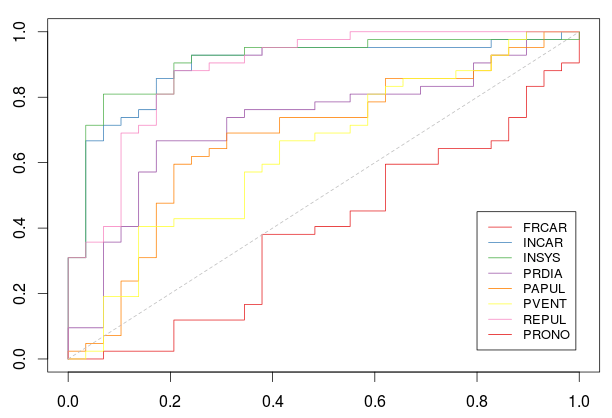with

```> AUC=rep(NA,7)
> for(k in 1:7){
+ AUC[k]=ROC(k)\$auc
+ cat("Variable ",k,"(",name_var[k],") :",
+ AUC[k],"n")
+ }
Variable  1 ( FRCAR ) : 0.3932677
Variable  2 ( INCAR ) : 0.8940887
Variable  3 ( INSYS ) : 0.908046
Variable  4 ( PRDIA ) : 0.7278325
Variable  5 ( PAPUL ) : 0.6756979
Variable  6 ( PVENT ) : 0.63711
Variable  7 ( REPUL ) : 0.8834154```

So, this time, INSYS is probably the best covariate to use. Now, if we keep that variable, and move forward,

```> k0=which.max(AUC)

> pred_i=function(i,k){
+  vk=c(k0,k)
+ fml = paste(name_var,"~",paste(name_var[vk],
+ collapse="+"),sep="")
+ reg=glm(fml,data=MYOCARDE[-i,],family=binomial)
+ predict(reg,newdata=MYOCARDE[i,],
+ type="response")
+ }
> library(AUC)
> ROC=function(k){
+   Y=MYOCARDE[,8]=="Survival"
+   L=list()
+   n=length(Y)
+   nk=trunc(n/trunc(n/10))
+   for(i in 1:(nk-1)) L[[i]]=((i-1)*
+     trunc(n/10)+1:(n/10))
+   L[[nk]]=((nk-1)*trunc(n/10)+1):n
+   S=unlist(Vectorize(function(i)
+      pred_i(L[[i]],k))(1:nk))
+   R=roc(S,as.factor(Y))
+   return(list(roc=cbind(R\$fpr,R\$tpr),
+               auc=AUC::auc(R)))
+ }

> plot(0:1,0:1,col="white",xlab="",ylab="")
> for(k in (1:7)[-k0])
+ lines(ROC(k)\$roc,col=CL[k])
> segments(0,0,1,1,lty=2,col="grey")
> legend(.8,.45,name_var[-k0],
+        col=CL[-k0],lty=1,cex=.8)```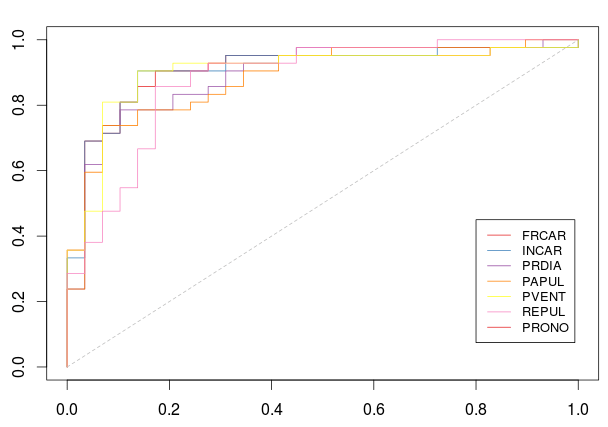and our best choice for the second variable would be INCAR

```> AUC=rep(NA,7)
> for(k in (1:7)[-k0]){
+   AUC[k]=ROC(k)\$auc
+   cat("Variable ",k,"(",name_var[k],") :",
+ AUC[k],"n")
+ }
Variable  1 ( FRCAR ) : 0.9047619
Variable  2 ( INCAR ) : 0.907225
Variable  4 ( PRDIA ) : 0.8916256
Variable  5 ( PAPUL ) : 0.8817734
Variable  6 ( PVENT ) : 0.9014778
Variable  7 ( REPUL ) : 0.8768473
> which.max(AUC)
 2```

Here again, it is possible to look at all pairs

```> pred_i=function(i,k){
+ fml = paste(name_var,"~",paste(name_var[
+ as.integer(k)],collapse="+"),sep="")
+ reg=glm(fml,data=MYOCARDE[-i,],family=binomial)
+ predict(reg,newdata=MYOCARDE[i,],
+ type="response")
+ }
> library(AUC)
> ROC=function(k){
+   Y=MYOCARDE[,8]=="Survival"
+   L=list()
+   n=length(Y)
+   nk=trunc(n/trunc(n/10))
+   for(i in 1:(nk-1)) L[[i]]=((i-1)*
+   trunc(n/10)+1:(n/10))
+   L[[nk]]=((nk-1)*trunc(n/10)+1):n
+   S=unlist(Vectorize(function(i)
+     pred_i(L[[i]],k))(1:nk))
+   R=roc(S,as.factor(Y))
+   return(AUC::auc(R))
+ }

> v=data.frame(k1=rep(1:7,each=7),k2=rep(1:7,7))
> v=v[v\$k1<v\$k2,]
> v\$auc=NA
> for(i in 1:nrow(v)) v\$auc[i]=ROC(v[i,1:2])
> v[which.max(v\$auc),]
k1 k2      auc
11  2  4 0.908867
> name_var[as.integer(v[which.max(v\$auc),1:2])]
 "INCAR" "PRDIA"```

which is the same as what we got using an One-Leave-Out strategy: we have again the same two covariates. And again, the AUC is lower than the one we got using a stepwise procedure (I still don’t understand how this is possible).   An alternative for the code would be to store all the regression models in a list,

```> L=list()
> n=nrow(MYOCARDE)
> nk=trunc(n/trunc(n/10))
> for(i in 1:(nk-1)) L[[i]]=((i-1)*trunc(n/10)+
+  1:(n/10))
> L[[nk]]=((nk-1)*trunc(n/10)+1):n
> REG=list()
> for(k in 1:7){
+   REG[[k]]=list()
+   fml = paste(name_var,"~",
+ paste(name_var[as.integer(k)],collapse="+"),
+ sep="")
+ for(i in 1:nk) REG[[k]][[i]]=reg=glm(fml,
+  data=MYOCARDE[-L[[i]],],family=binomial)
+ }```

and then to call them, properly

```> pred_i=function(i,k){
+  I=which(sapply(1:10,function(j) i%in%L[[j]]))
+  predict(REG[[k]][[I]],newdata=MYOCARDE[i,],
+  type="response")
+ }```

One has to check about the efficiency, especially with a large dataset.

• Using Trees and Random Forests

Another quick, but popular (from what I’ve seen), technique is to use trees. Important variables should appear in the output,

```> library(rpart)
> tree=rpart(PRONO~.,data=MYOCARDE)
> library(rpart.plot)
> rpart.plot(tree)```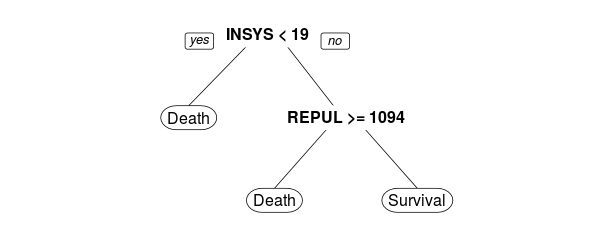Here, the first variable that appears in the tree construction is INSYS, and the second on is REPUL. Which is rather different with what we got above. But using one tree is maybe not sufficient. One can use the variable importance function (described in a previous post) obtained using random forests.

```> library(randomForest)
> rf=randomForest(PRONO~.,data=MYOCARDE,
+                 importance=TRUE)
> rf\$importance[,4]
FRCAR    INCAR    INSYS
1.042006 7.363255 8.954898
PRDIA    PAPUL    PVENT
3.149235 2.571267 3.152619
REPUL
7.510110```

Here, we have the same story as the one we got with a simple tree: the ‘most important’ variable is INSYS while the second one is REPUL. But here, we consider tree based predictors. And not a logistic regression.

• Using Dedicated R functions

It is possible to use some dedicated R functions for variable selection. For instance, since we consider a logistic regression, use

```> library(bestglm)
> Xy=as.data.frame(MYOCARDE)
> Xy[,8]=(Xy[,8]=="Death")*1
> names(Xy)=names(MYOCARDE)
> B=bestglm(Xy)
> B\$Subsets[,2:8]
FRCAR INCAR INSYS PRDIA PAPUL PVENT REPUL
0  FALSE FALSE FALSE FALSE FALSE FALSE FALSE
1  FALSE FALSE FALSE FALSE FALSE FALSE  TRUE
2* FALSE  TRUE FALSE FALSE FALSE FALSE  TRUE
3  FALSE  TRUE FALSE FALSE FALSE  TRUE  TRUE
4   TRUE  TRUE FALSE FALSE FALSE  TRUE  TRUE
5   TRUE  TRUE  TRUE FALSE FALSE  TRUE  TRUE
6   TRUE  TRUE FALSE  TRUE  TRUE  TRUE  TRUE
7   TRUE  TRUE  TRUE  TRUE  TRUE  TRUE  TRUE```

With only one variable, we should consider REPUL (row 1 of the matrix), while with two variables, we should consider REPUL and INCAR (and that is the best model, based on some Bayesian Information Criterion). Here, cross validation techniques can be used also,

```> B=bestglm(Xy, IC = "CV", CVArgs =
+ list(Method = "HTF", K = 10, REP = 1))
>  cverrs = B\$Subsets[, "CV"]
>  sdCV = B\$Subsets[, "sdCV"]
>  CVLo = cverrs - sdCV
>  CVHi = cverrs + sdCV
>  ymax = max(CVHi)
>  ymin = min(CVLo)
>  k = 0:(length(cverrs) - 1)
>  plot(k, cverrs, ylim = c(ymin,
+  ymax), type = "n", yaxt = "n")
>  points(k,cverrs,cex = 2,col="red",pch=16)
>  lines(k, cverrs, col = "red", lwd = 2)
>  axis(2, yaxp = c(0.6, 1.8, 6))
>  segments(k, CVLo, k, CVHi,col="blue", lwd = 2)
>  eps = 0.15
>  segments(k-eps, CVLo, k+eps, CVLo,
+ col = "blue", lwd = 2)
>  segments(k-eps, CVHi, k+eps, CVHi,
+ col = "blue", lwd = 2)
>  indMin = which.min(cverrs)
>  fmin = sdCV[indMin]
>  cutOff = fmin + cverrs[indMin]
>  abline(h = cutOff, lty = 2)
>  indMin = which.min(cverrs)
>  fmin = sdCV[indMin]
>  cutOff = fmin + cverrs[indMin]
>  min(which(cverrs < cutOff))
 2```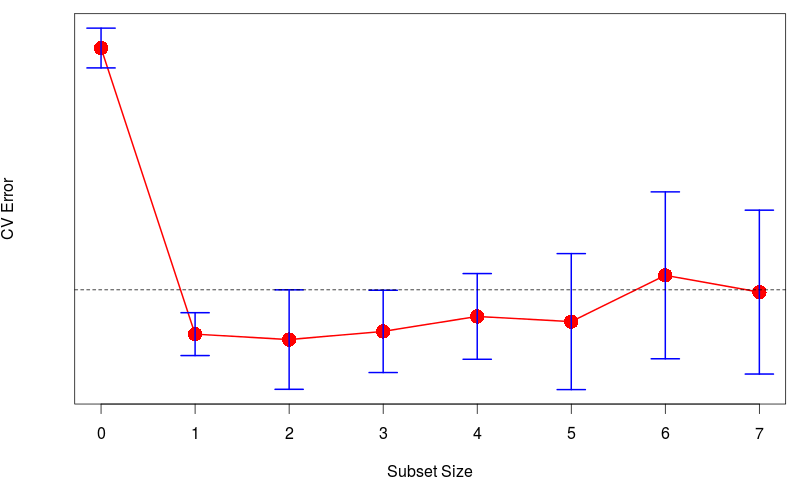If we summarize, here,

• stepwise, AIC : REPUL + INCAR
• stepwise, BIC : REPUL + INCAR
• One-leave-Out CV stepwise : REPUL + INCAR
• One-leave-Out CV pairs : INCARPRDIA
• -fold CV stepwise : INSYS + INCAR
• -fold CV pairs : INCARPRDIA
• Tree : INSYS + REPUL
• Variable Importance (RF) : INSYS + INCAR
• Best GLM : REPUL + INCAR

That is the lovely part with statistical tools: there are usually multiple (valid) answers. And this is why machine learning is difficult: if there was a single answer, any machine could built up a model that works well. But obviously, it has to be more complicated…